nick@virtualimage.co.ukNo reproduction of any sort by any means are allowed without written authorization from Nicholas Mee.

nick@virtualimage.co.ukHiggs Force: QEDIn 1894, the Scottish physicist C. T. R. Wilson spent his vacation at a weather station on the summit of Ben Nevis. While on the mountain, Wilson witnessed a ghostly optical apparition known as the Brocken spectre.The Brocken spectre. (Copyright Michael Yakobi)Back in the Cavendish Laboratory in Cambridge, Wilson set out to reproduce the atmospheric effects that had created the illusion. The culmination of this research was the cloud chamber, an instrument that would show the tracks of charge particles passing through it. This device would play a vital role in the discovery of the electron by Wilson’s boss, the director of the Cavendish Laboratory J. J. Thomson. The cloud chamber also had an important part to play in the career of another Cambridge physicist Paul Dirac, one of the great theoretical physicists of the 20th century. Dirac had an intuitive feeling that the correct description of fundamental physics must be simple and elegant. Symmetry lay at the heart of his mathematics, which always had a beautiful austerity. When Dirac was asked to summarise his philosophy of physics, he wrote a single sentence on the blackboard: PHYSICAL LAWS SHOULD HAVE MATHEMATICAL BEAUTY One night in 1928 Dirac sat in his room in St John’s College staring into the fire. As he let his mind drift amidst the flames, he was struck by the idea that would enable him to construct an equation of a completely novel kind. He could see at once that his new approach was going to work. The equation describes the evolution of matter waves, such as electron waves, even when their speed approaches the speed of light. Dirac’s analysis of his equation persuaded him that there was a previously unsuspected symmetry at the heart of matter. The equation only made sense if the electron had a mirror image particle. A particle whose mass was the same as the mass of the electron, but whose electric charge was positive instead of negative. Just four year’s later the particle was found by an American physicist Carl Anderson who had constructed a cloud chamber in which he could study the tracks left by cosmic rays. We know this particle, the antiparticle of the electron, as the positron. But Dirac’s equation describes the behaviour of all the different types of particles from which matter is formed. So it follows that there must also be a mirror image particle for other particles, such as the proton and the neutron. This is indeed the case. The antiproton was found in 1955 and the antineutron in 1956. These antiparticles will combine to form antimatter in the same way that ordinary particles combine to form matter. For example, a positron will bind to an antiproton to form an atom of antihydrogen. These antiatoms are now being actively studied in the laboratory at CERN. Dirac’s interpretation of his equation offered a deep insight into electromagnetic interactions at the level of fundamental particles. According to Dirac, when an electron and a positron meet they can mutually annihilate and disappear in a burst of high energy gamma ray photons. Conversely, it is possible for a gamma ray photon to convert into an electron and a positron. This was a completely new idea. Matter was not eternal, it could be created or destroyed. Dirac had been led to formulate his equation by the desire to make quantum mechanics compatible with Einstein’s relativity. And now the inter-convertibility of matter and radiation that Dirac had deduced from his equation gave a new significance to the most famous equation that Einstein had derived from his theory: E = mc² . This equation sums up the equivalence of mass and energy in a statement that is simple enough to be used as a slogan on a tee-shirt. Dirac had now shown how the conversion of mass into energy and energy into mass worked at the level of particle interactions. Dirac had taken the first step towards a quantum theory of electromagnetism. In the pictures drawn by Michael Faraday a charged particle such as an electron is surrounded by an electric field that permeates the whole of space. This is just a map of the electric force around the electron. In the new quantum picture of Faraday’s electric and magnetic fields, the fields are not continuous throughout space, they are composed of photons. The electron is bathed in a fluctuating cloud of photons that it is continually emitting and reabsorbing. This cloud of photons, which corresponds to Faraday’s electromagnetic field, is responsible for producing the electromagnetic force between charged particles. The way this works is that a photon that has been emitted by one electron might be absorbed by a second nearby electron. The photon has no way of recognizing its parent electron from any other electron so this process will inevitably happen to some of the photons in the cloud. The emission of the photon gives a kick to the first electron and its absorption gives an oppositely directed kick to the second electron. In this way the exchanged photon has transferred some of the energy and momentum of the first electron to the second electron. The trajectory of both electrons will be altered. This is the way that forces operate in quantum theory. They are due to the interaction of individual particles. The electromagnetic force is a consequence of the exchange of photons between charged particles. This is a nice picture, but physicists need to be able to perform calculations in order to test a theory against experimental results. Unfortunately, there were serious technical difficulties in performing these calculations that would not be ironed out until after the Second World War. Several theorists contributed to the resolution of these difficulties, but the most elegant methods were found by a charismatic New Yorker called Richard Feynman. He was a great puzzle solver, who couldn’t sleep when introduced to a new puzzle until he had it cracked. Feynman liked to think visually and while playing with the equations of quantum electrodynamics (QED) he developed a pictorial shorthand to help him keep track of all the terms in the horrendously complicated equations. Each diagram was a kind of glyph that represented a mathematical expression, where each part of the diagram corresponded to a mathematical term that formed part of the expression. Feynman devised a recipe that allowed him to translate back and forth between the mathematical expressions that cropped up in QED calculations and his diagrams. This was very useful, because it is much easier to remember the structure of a simple diagram than the exact form of a complicated mathematical expression. But Feynman soon realised that his diagrams were much more than simply mnemonics. Not only did each picture represent a mathematical expression, it also appeared to depict a physical process – a type of particle interaction. The first diagram that Feynman published in 1948 is shown below. It depicts an interaction between two electrons that is mediated by the exchange of a photon between them. The two electrons are represented by lines with arrows on them and the photon is represented by the wavy line. Time runs upwards in the diagram, which shows the two electrons approach each other, exchange a photon and then retreat from each other.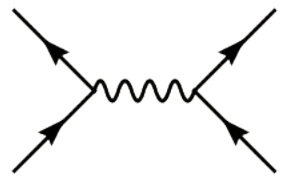Although the diagram is only a schematic representation of an interaction between two particles, it clearly looks very much like the scattering event described above. But the diagram also corresponds to the first of many mathematical expressions that pop out of the QED calculations. Feynman realised that each expression can be represented by a similar diagram. He then showed that by thinking in terms of his diagrams all the terms that were needed for a QED calculation could be found without the need for any of the complicated and tedious algebraic manipulation that had previously been required. All that a theorist had to do was to construct all the possible diagrams. Then, using Feynman’s recipe, each diagram could be converted into the appropriate mathematical expression. This process eliminated pages of arduous algebraic hieroglyphics in which slips would almost certainly creep in for even the most meticulous theoretical physicist. To complete a calculation it was still necessary to evaluate the mathematical expression corresponding to each diagram and to perform an exact calculation, all possible diagrams would have to be included. But there is another nice feature of the diagrams. The simpler diagrams give much bigger contributions to the final answer than the complicated diagrams. They are also much easier to evaluate. It is possible to get a very accurate answer even if all the complicated diagrams are ignored. The enormous power of Feynman diagrams can be illustrated by what is surely the most amazing and involved calculation ever performed. The motion of the electric charge of an electron as it spins produces a tiny magnetic field that is intrinsic to the electron. It is known as the magnetic moment of the electron. One of the great successes of Dirac’s equation was that it predicted that the magnetic moment of the electron is exactly 2 in the appropriate units. This figure is very close to the measured value, so it was seen as a great success when it was first derived. But when more accurate measurements became possible it was clear that there was a very small descrepancy and that the correct figure was slightly more than 2. The technique pioneered by Feynman can be used to improve the calculation. The simplest Feynman diagram that is relevant to this calculation is shown below. The line with the arrow on it represents an electron and the wavy line is a photon that forms part of the magnetic field of the electron. This photon is emitted by the electron and is picked up by our measuring apparatus. When the diagram is evaluated it is equal to 2, corresponding to the value for the electron’s magnetic moment given by Dirac’s equation.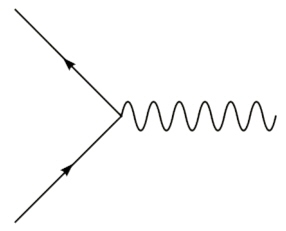But the accuracy of the calculation can be improved by evaluating further diagrams. The next simplest diagram is shown below. It has a single loop. This diagram represents a process in which the electron emits a photon before its magnetic field interacts with our measuring equipment. The photon is then reabsorbed by the electron.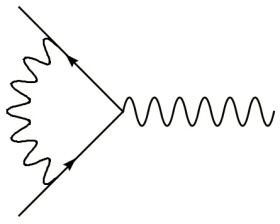By evaluating diagrams with increasing numbers of loops it is possible to approach ever closer to the total value of the magnetic moment. But at each step there are more diagrams and the effort involved in evaluating each one of them greatly increases. There is just one diagram with a single loop, but there are 7 diagrams with two loops and 72 diagrams with three loops and a daunting 891 four-loop diagrams. But as we have noted, the more loops that a diagram contains the smaller its contribution to the final answer will be. The one loop diagram gives a contribution to the full answer that is about one thousandth of the total. The sum of the two loop diagrams will be around one millionth of the total. The sum of the three loop diagrams will give of the order of a billionth of the total and the sum of the four loop diagrams contributes just a trillionth to the final answer. Two examples of the four-loop diagrams are shown below. In principle, we can calculate the value of the magnetic moment of the electron to whatever precision we like, as long as we have sufficient patience to evaluate the diagrams.And there are members of the physics community that do have the incredible patience required to perform this calculation to the precision of a few parts in a trillion. The QED calculation has now been underway for around 60 years and the latest theoretical value for the magnetic moment of the electron includes contributions from a total of about one thousand Feynman diagrams - all the diagrams with up to four loops. The result of the calculation is that the electron’s magnetic moment is predicted to be equal to: 2 x (1.001 159 652 178). The illustration shows the level at which the contribution to this total from each set of diagrams kicks in.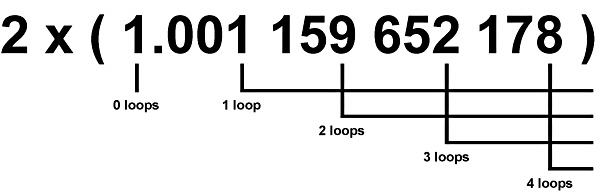Experimental techniques for measuring the electron’s magnetic moment have advanced in step with the progress in the theoretical calculation. The most recent experimental determination of the magnetic moment of the electron was published by a group at Harvard University in 2006 and it agrees with the theoretical value exactly. To within an accuracy of less than 10 parts in a trillion the prediction of QED has been confirmed. This is an absolutely exquisite agreement between theory and experiment. The level of precision is equivalent to the measurement of a distance equal to the Earth’s circumference with an accuracy within the width of a very fine human hair. But not only that, the position of the hair would not simply have to be measured to this accuracy, its position would have to be predicted to this accuracy beforehand. This represents the most profound agreement between theory and experiment ever achieved. There is no indication from this or any other experiment that QED has been stretched beyond its range of applicability. This is certainly one of the great triumphs of modern science.www.higgsforce.co.uk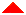Home   Particle Physics   Science and Art   Geometry   Cryptography   Mathematical Games   Educational Software   Gallery   SupersymmetryCopyright © 2019 Nicholas Mee. All Rights Reserved.